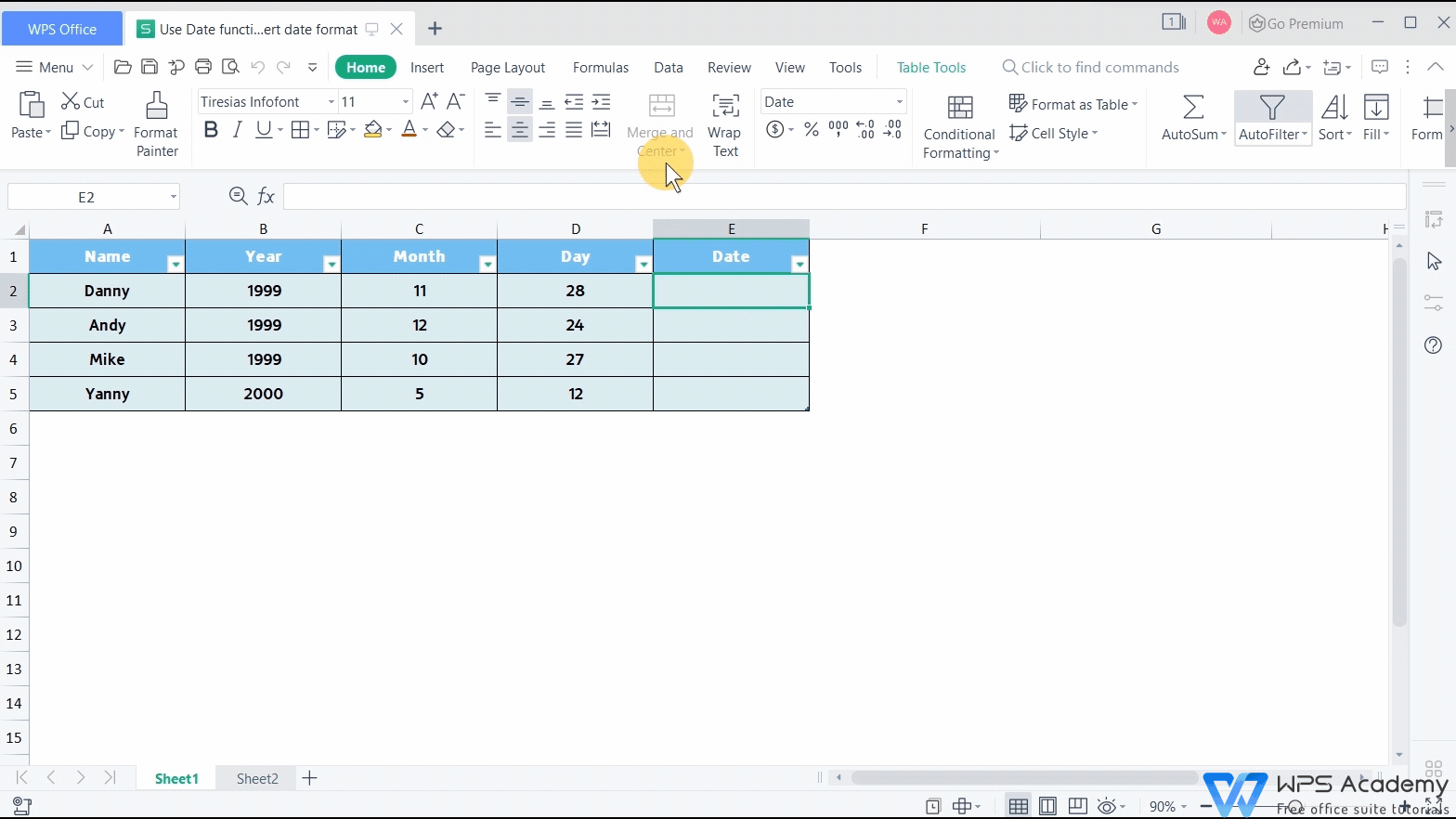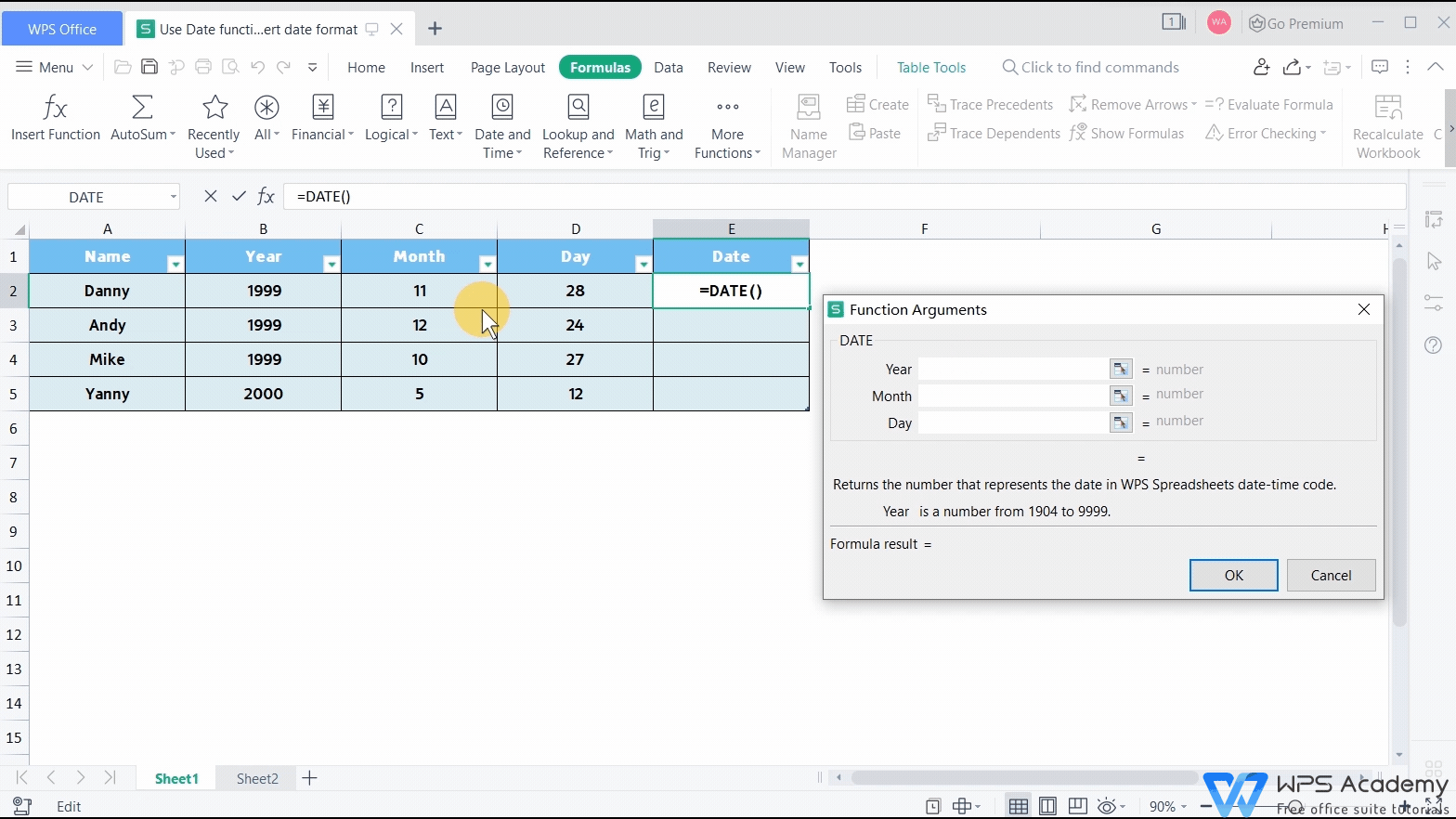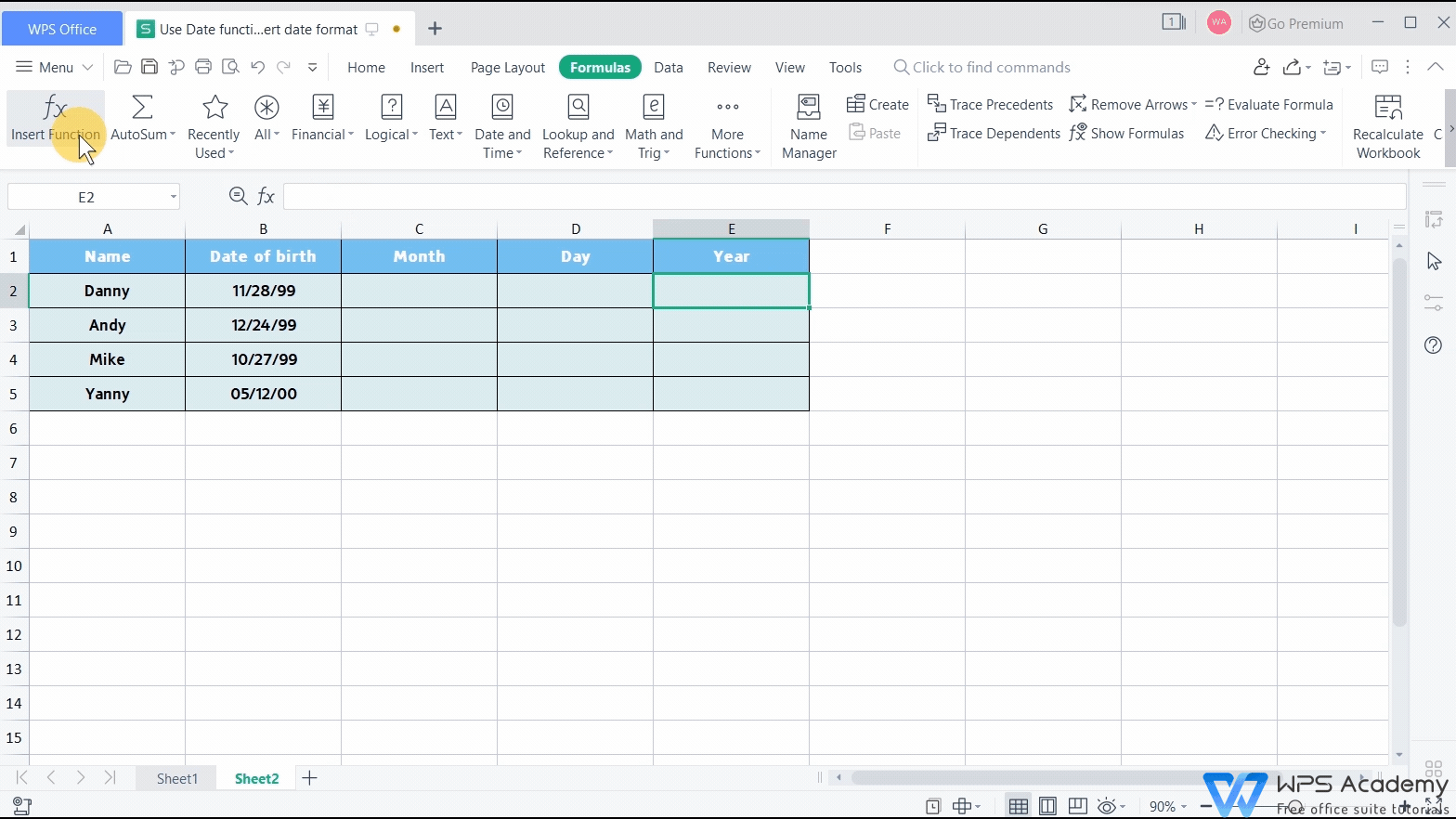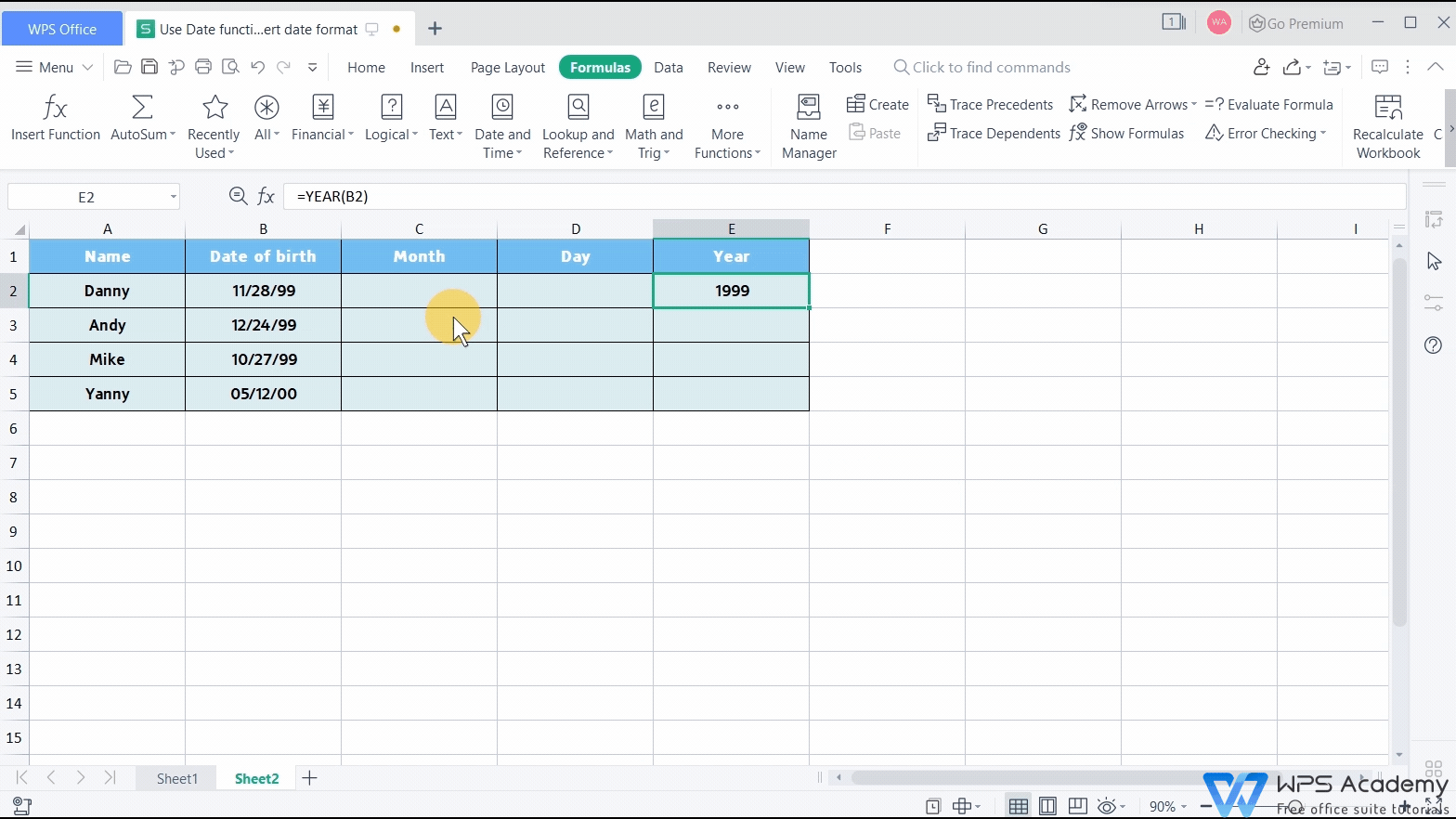# Use Date function to convert date format### Graphic skills

We may sometimes need to convert the format of the date in the table when using WPS Spreadsheet like Microsoft Excel. At this point, by using the Date function, we can quickly generate a specific date symbol.

Take this table as an example. This table contains the information on the date of birth of some students in class. We can see that the information of year, month, and day are separated in this table. So how can we convert the format quickly to know the birthdays of the students?

1. First, select cell E2 to insert the Date function, and click OK.2. Select the year, month, and day cells respectively in the pop-up Function Argument dialog box. For example, we select B2 for Year, C2 for Month, D2 for Day.

3. Then click OK. Now we can see that the date has converted to a common format.Conversely, if we want to split the date, we can use the YEAR function, the MONTH function, and the DAY function.

1. Select cell E2. Insert the YEAR function, and enter B2 in serial_number.

2. Then click OK. We can see that the Year has been extracted from the Date of Birth in cell B2.We can also use the MONTH function and the DAY function to extract the Month and Year from cell B2 in the same way. Finally, select the table area and use the shortcut key Ctrl+D to fill cells.What a practical feature on DATE funtion!

Did you get it?